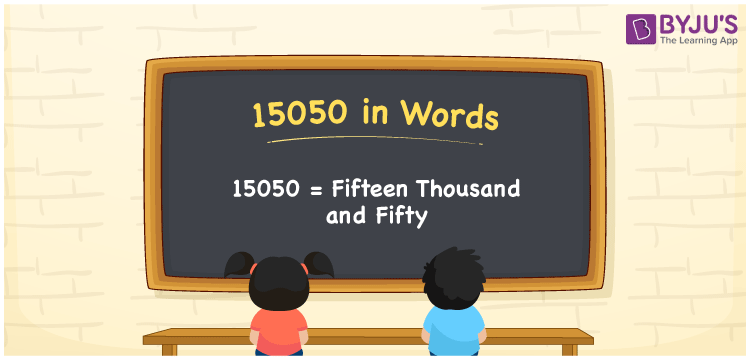# 15050 in words

15050 in words is written as Fifteen Thousand and Fifty. In 15050, the 1 has a place value of ten thousand, first 5 is in the place value of thousand and the next 5 is in the place value of ten. The article on Place Value gives more information. The number 15050 is used in expressions that relate to money, distance, social media views, and many more. For example, “The monthly school fee is Fifteen Thousand and Fifty.”

 15050 in words Fifteen Thousand and Fifty Fifteen Thousand and Fifty in Numbers 15050

## 15050 in English Words## How to Write 15050 in Words?

We can convert 15050 to words using a place value chart. The number 15050 has 5 digits, so let’s make a chart that shows the place value up to 5 digits.

 Ten thousand Thousands Hundreds Tens Ones 1 5 0 5 0

Thus, we can write the expanded form as:

1 × Ten thousand + 5 × Thousand + 0 × Hundred + 5 × Ten + 0 × One

= 1 × 10000 + 5 × 1000 + 0 × 100 + 5 × 10 + 0 × 1

= 15050.

= Fifteen Thousand and Fifty.

15050 is the natural number that is succeeded by 15049 and preceded by 15051.

15050 in words – Fifteen Thousand and Fifty.

Is 15050 an odd number? – No.

Is 15050 an even number? – Yes.

Is 15050 a perfect square number? – No.

Is 15050 a perfect cube number? – No.

Is 15050 a prime number? – No.

Is 15050 a composite number? – Yes.

## Solved Example

1. Write the number 15050 in expanded form

Solution: 1 x 10000 + 5 x 1000 + 0 x 100 + 5 x 10 + 0 x 1

We can write 15050 = 10000 + 5000 + 000 + 50 + 0

= 1 x 10000 + 5 x 1000 + 0 x 100 + 5 x 10 + 0 x 1.

## Frequently Asked Questions on 15050 in words

### How to write the number 15050 in words?

15050 in words is written as Fifteen Thousand and Fifty.

### Is 15050 a prime number?

No. 15050 is not a prime number.

### Is 15050 divisible by 10?

Yes. 15050 is divisible by 10.